Скачать презентацию Foundations of Multinational Financial Management Alan Shapiro J

2eacf7ae925ba48f4846bcc772f2d473.ppt

• Количество слайдов: 34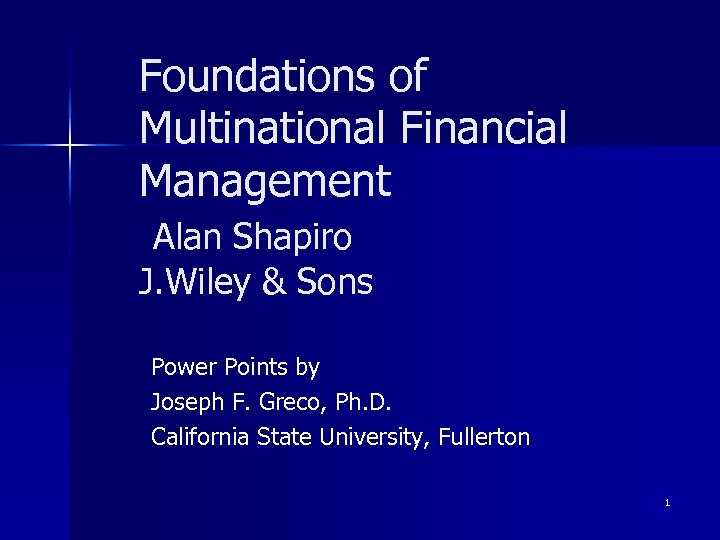Foundations of Multinational Financial Management Alan Shapiro J. Wiley & Sons Power Points by Joseph F. Greco, Ph. D. California State University, Fullerton 1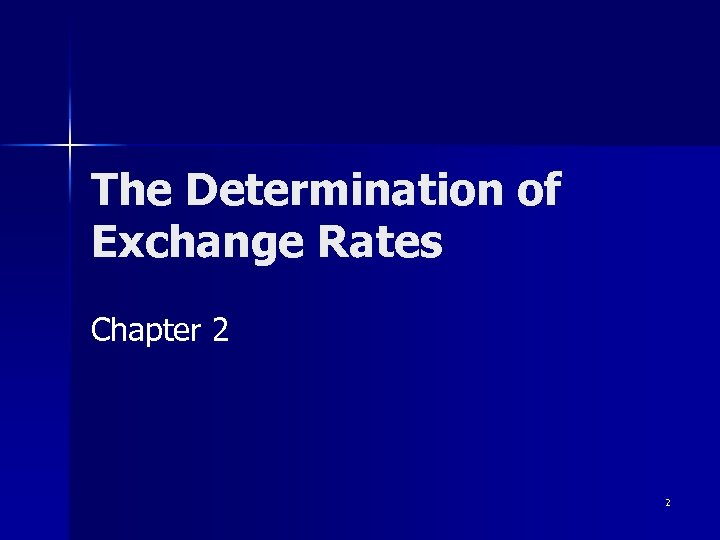The Determination of Exchange Rates Chapter 2 2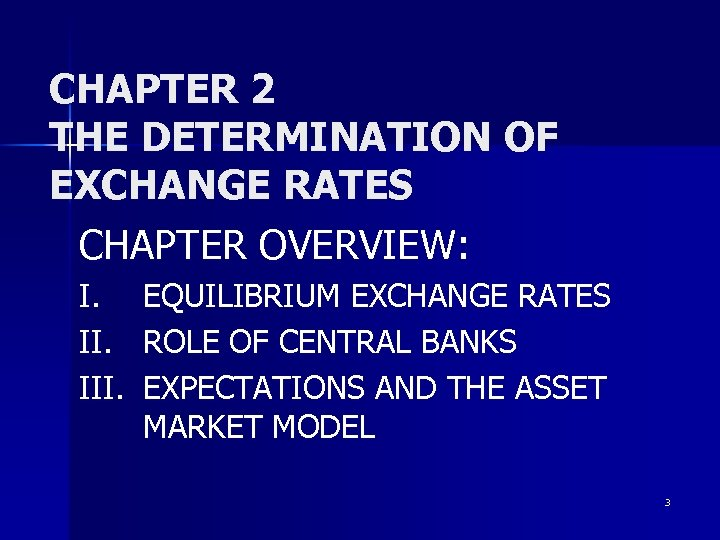CHAPTER 2 THE DETERMINATION OF EXCHANGE RATES CHAPTER OVERVIEW: I. III. EQUILIBRIUM EXCHANGE RATES ROLE OF CENTRAL BANKS EXPECTATIONS AND THE ASSET MARKET MODEL 3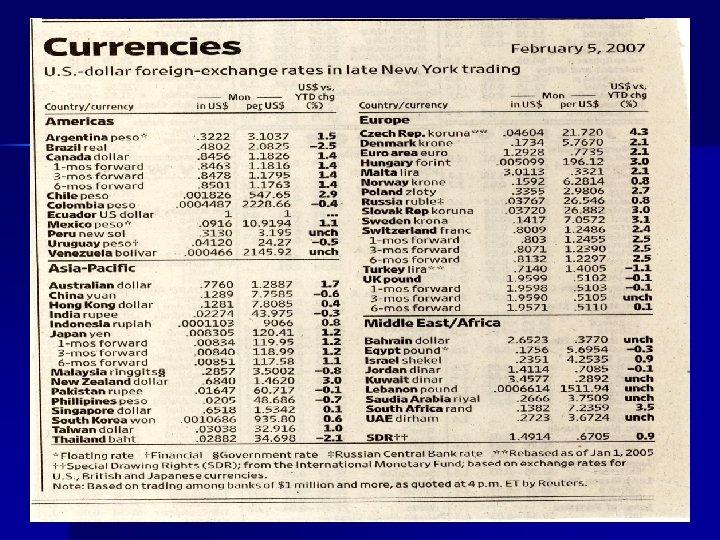4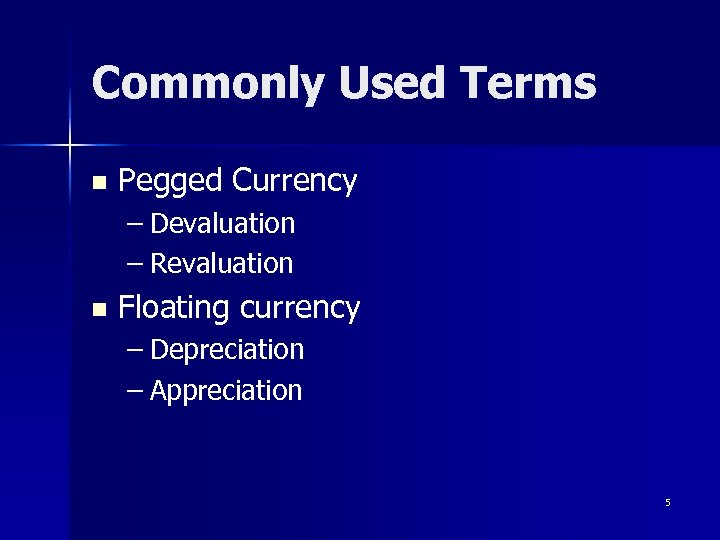Commonly Used Terms n Pegged Currency – Devaluation – Revaluation n Floating currency – Depreciation – Appreciation 5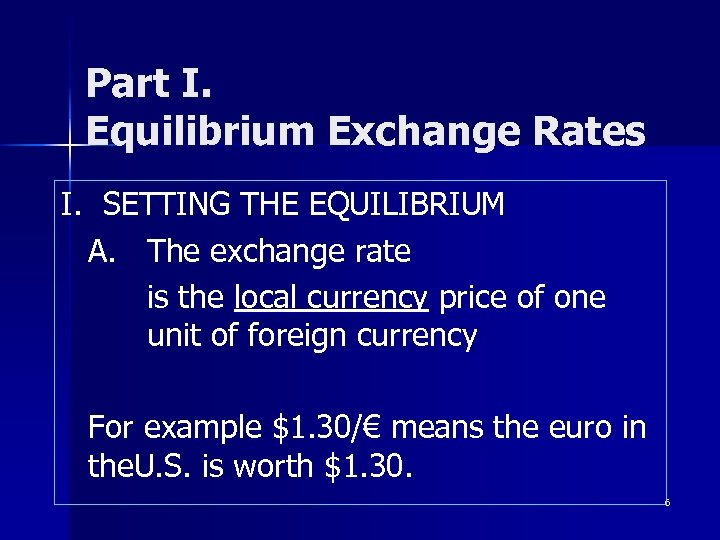Part I. Equilibrium Exchange Rates I. SETTING THE EQUILIBRIUM A. The exchange rate is the local currency price of one unit of foreign currency For example \$1. 30/€ means the euro in the. U. S. is worth \$1. 30. 6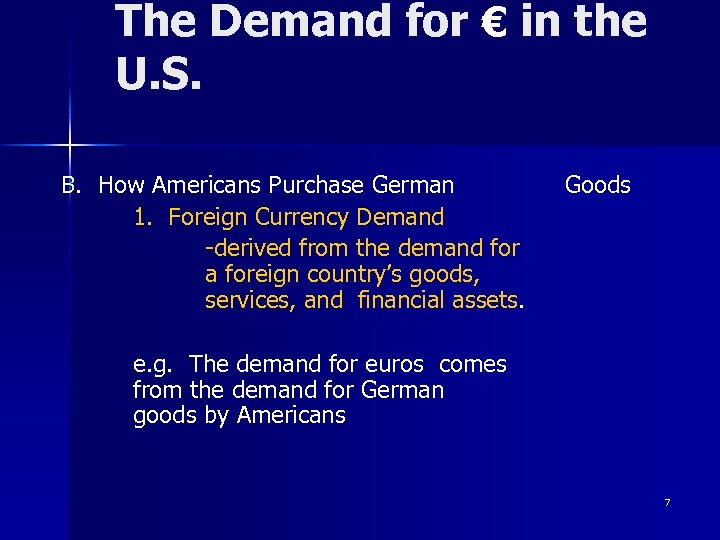The Demand for € in the U. S. B. How Americans Purchase German 1. Foreign Currency Demand -derived from the demand for a foreign country’s goods, services, and financial assets. Goods e. g. The demand for euros comes from the demand for German goods by Americans 7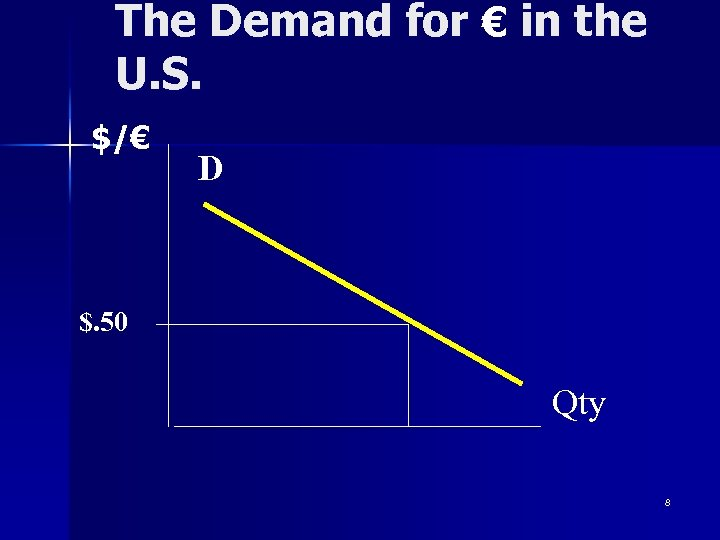The Demand for € in the U. S. \$/€ D \$. 50 Qty 8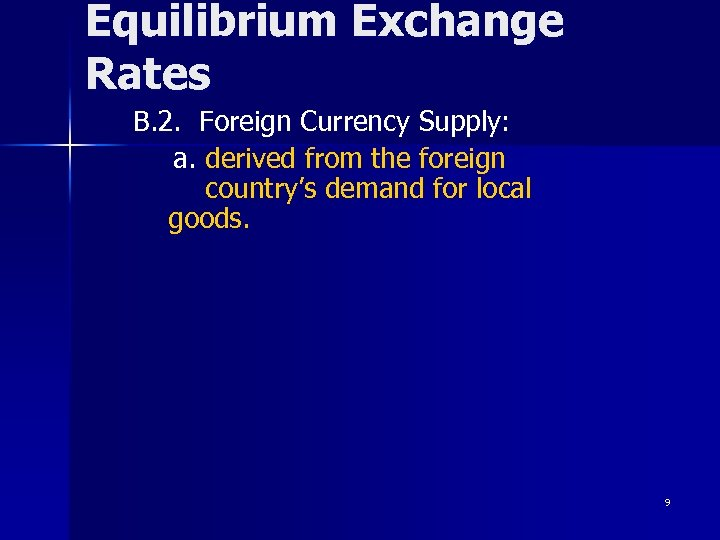Equilibrium Exchange Rates B. 2. Foreign Currency Supply: a. derived from the foreign country’s demand for local goods. 9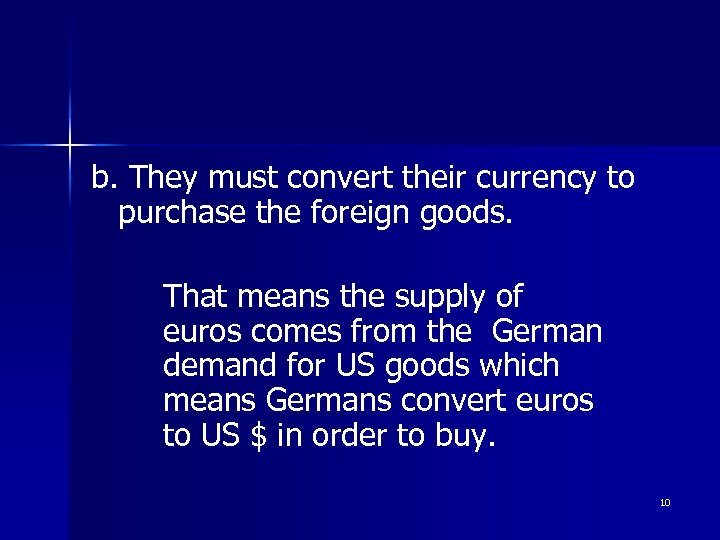b. They must convert their currency to purchase the foreign goods. That means the supply of euros comes from the German demand for US goods which means Germans convert euros to US \$ in order to buy. 10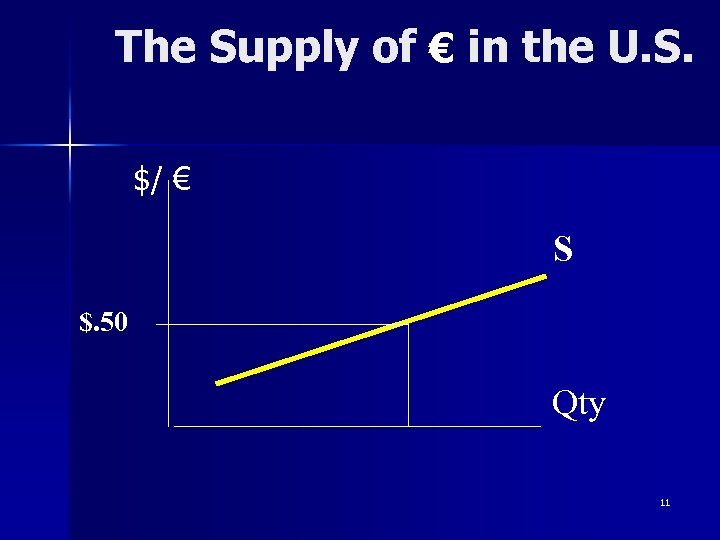The Supply of € in the U. S. \$/ € S \$. 50 Qty 11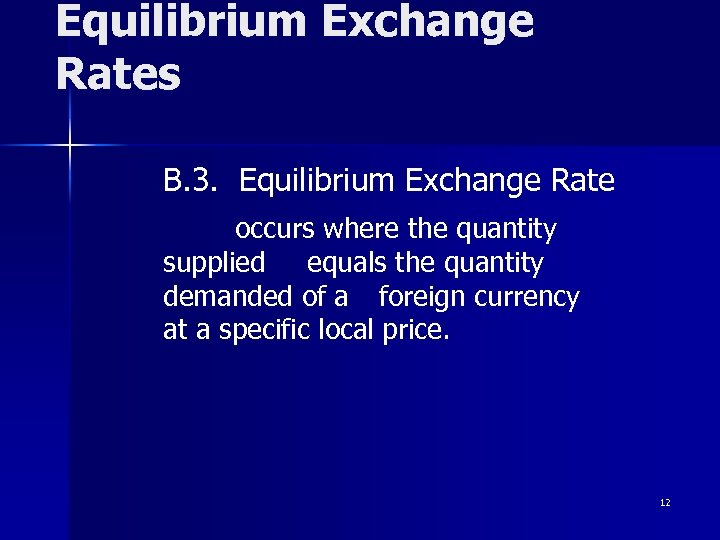Equilibrium Exchange Rates B. 3. Equilibrium Exchange Rate occurs where the quantity supplied equals the quantity demanded of a foreign currency at a specific local price. 12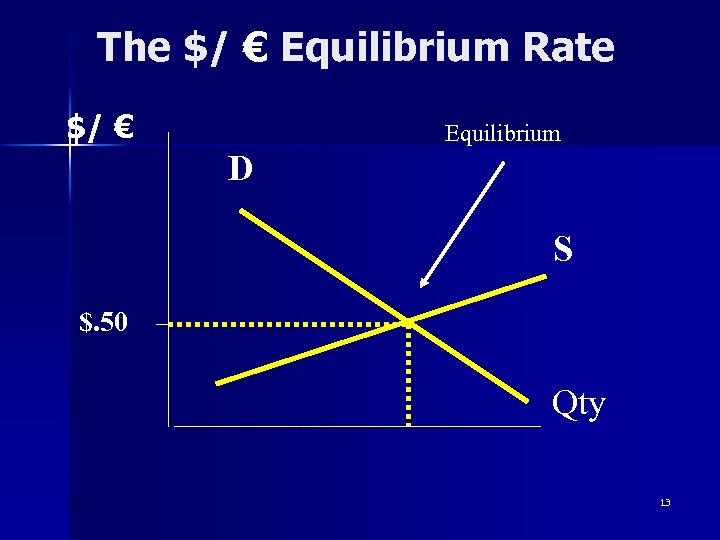The \$/ € Equilibrium Rate \$/ € Equilibrium D S \$. 50 Qty 13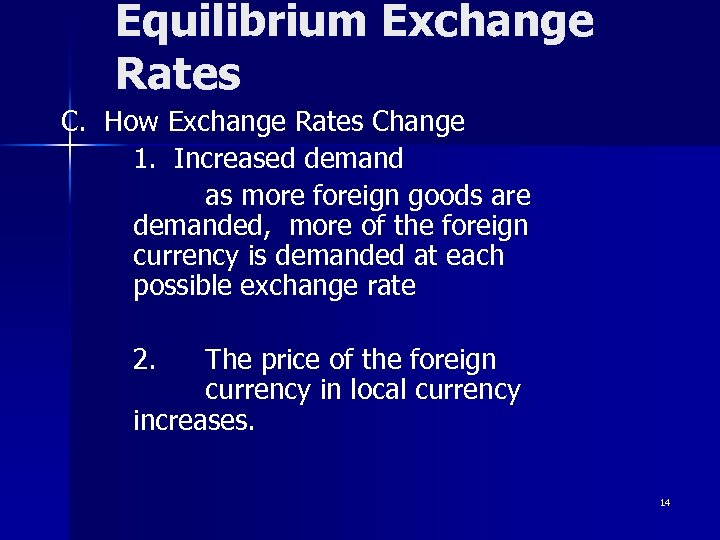Equilibrium Exchange Rates C. How Exchange Rates Change 1. Increased demand as more foreign goods are demanded, more of the foreign currency is demanded at each possible exchange rate 2. The price of the foreign currency in local currency increases. 14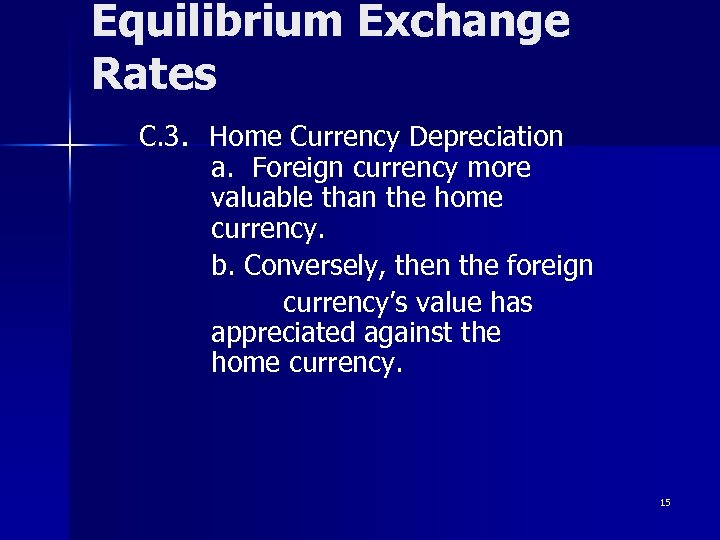Equilibrium Exchange Rates C. 3. Home Currency Depreciation a. Foreign currency more valuable than the home currency. b. Conversely, then the foreign currency’s value has appreciated against the home currency. 15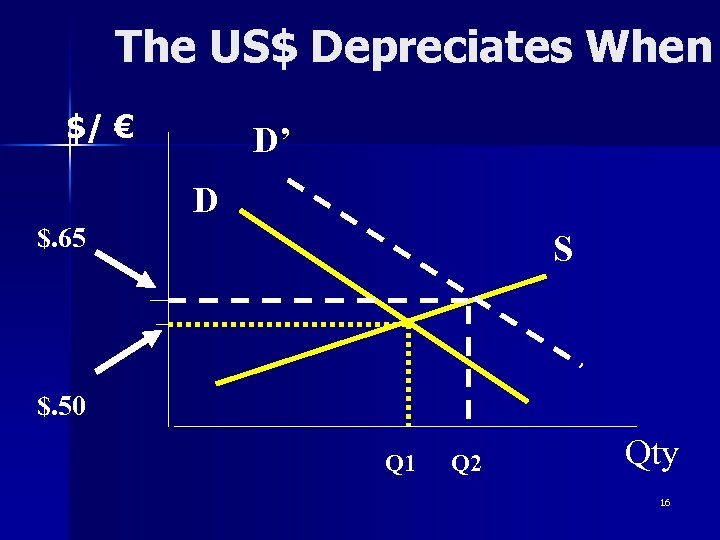The US\$ Depreciates When \$/ € D’ D \$. 65 S \$. 50 Q 1 Q 2 Qty 16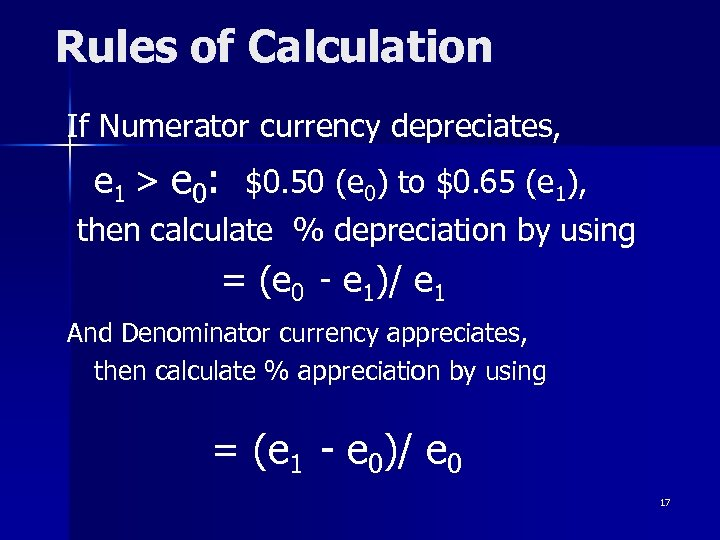Rules of Calculation If Numerator currency depreciates, e 1 > e 0: \$0. 50 (e 0) to \$0. 65 (e 1), then calculate % depreciation by using = (e 0 - e 1)/ e 1 And Denominator currency appreciates, then calculate % appreciation by using = (e 1 - e 0)/ e 0 17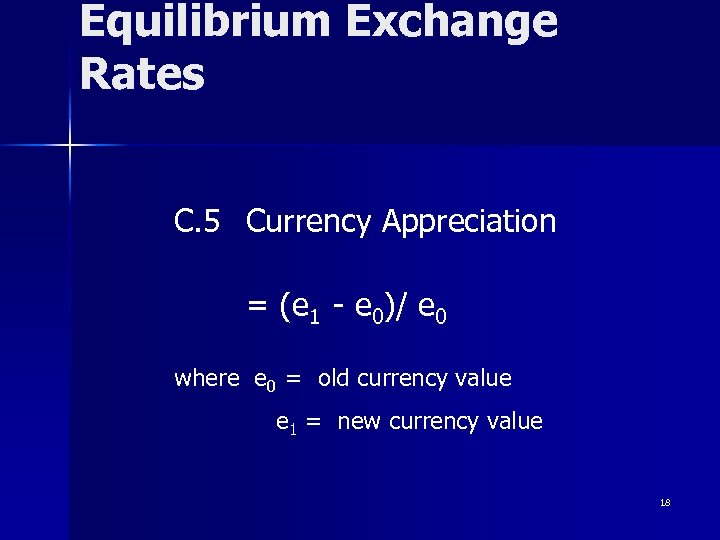Equilibrium Exchange Rates C. 5 Currency Appreciation = (e 1 - e 0)/ e 0 where e 0 = old currency value e 1 = new currency value 18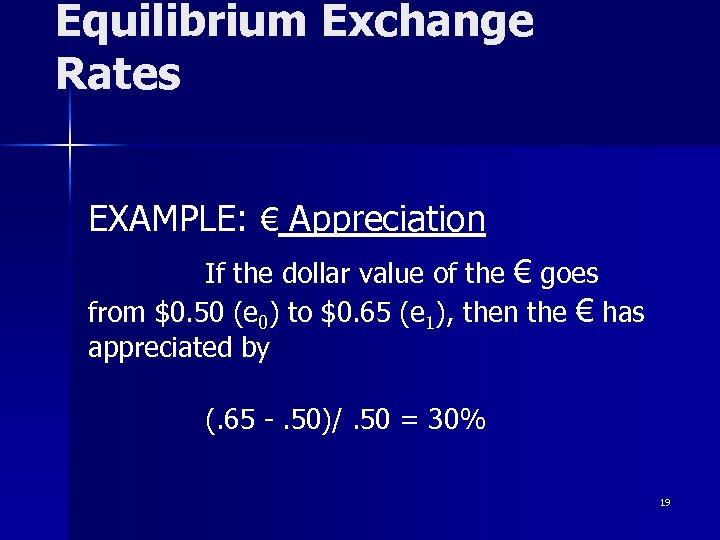Equilibrium Exchange Rates EXAMPLE: € Appreciation If the dollar value of the € goes from \$0. 50 (e 0) to \$0. 65 (e 1), then the € has appreciated by (. 65 -. 50)/. 50 = 30% 19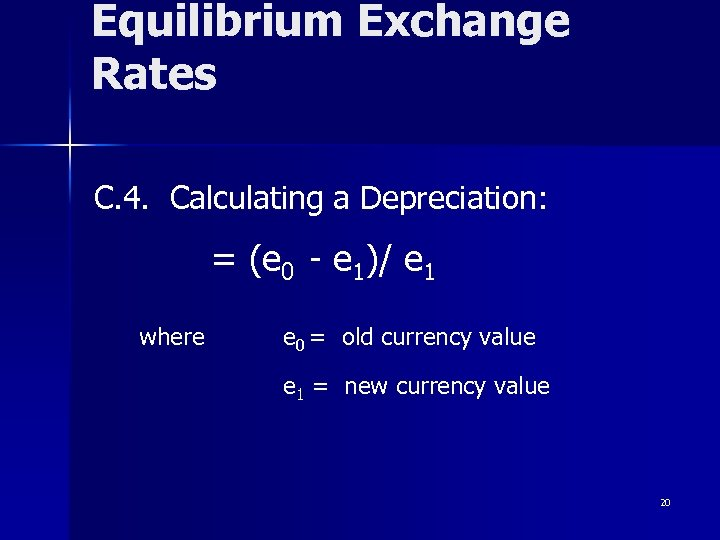Equilibrium Exchange Rates C. 4. Calculating a Depreciation: = (e 0 - e 1)/ e 1 where e 0 = old currency value e 1 = new currency value 20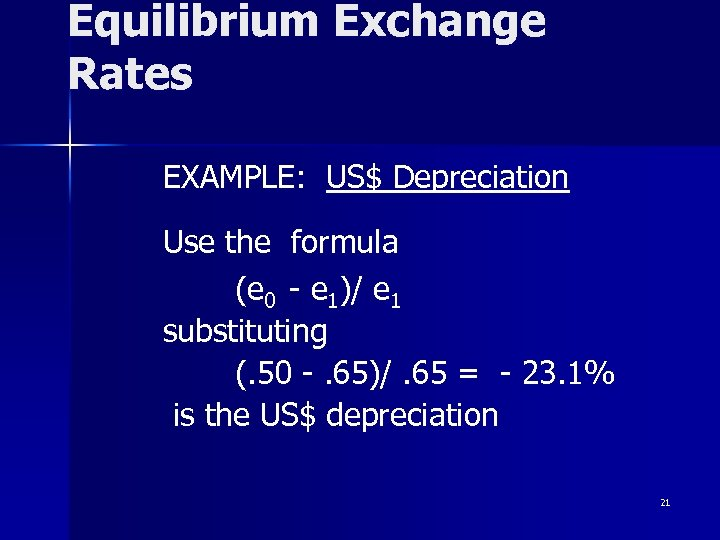Equilibrium Exchange Rates EXAMPLE: US\$ Depreciation Use the formula (e 0 - e 1)/ e 1 substituting (. 50 -. 65)/. 65 = - 23. 1% is the US\$ depreciation 21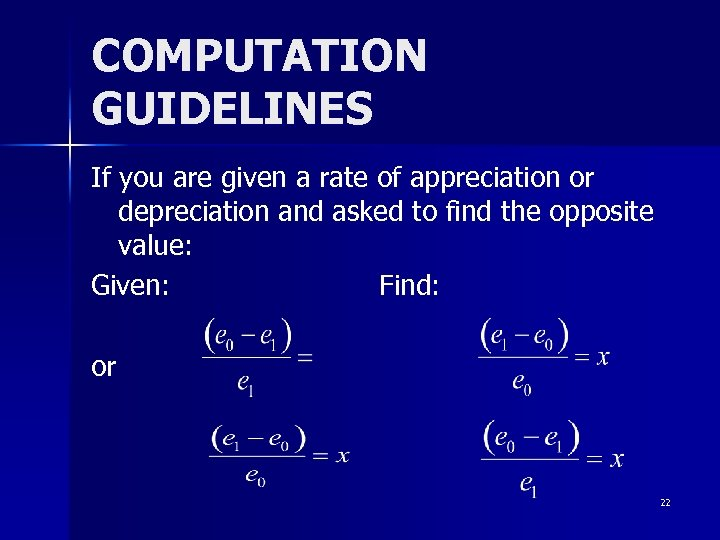COMPUTATION GUIDELINES If you are given a rate of appreciation or depreciation and asked to find the opposite value: Given: Find: or 22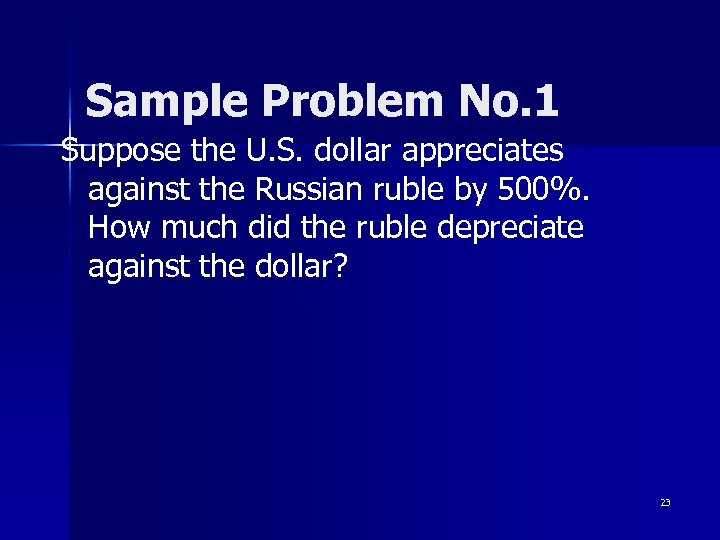Sample Problem No. 1 Suppose the U. S. dollar appreciates against the Russian ruble by 500%. How much did the ruble depreciate against the dollar? 23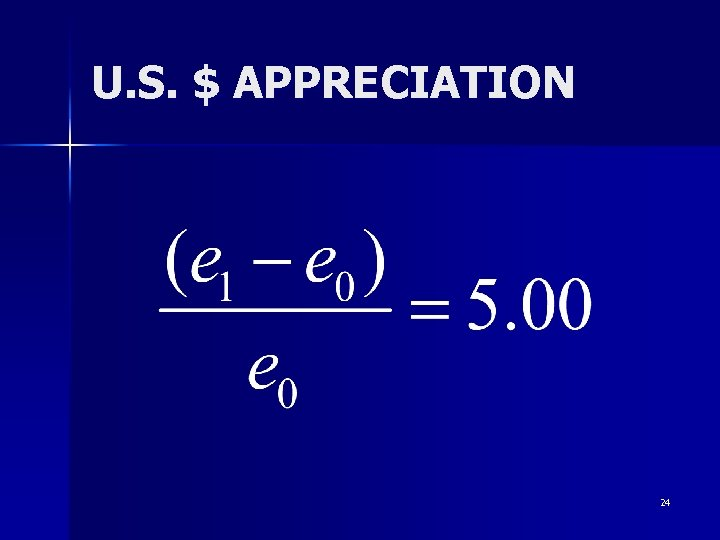U. S. \$ APPRECIATION 24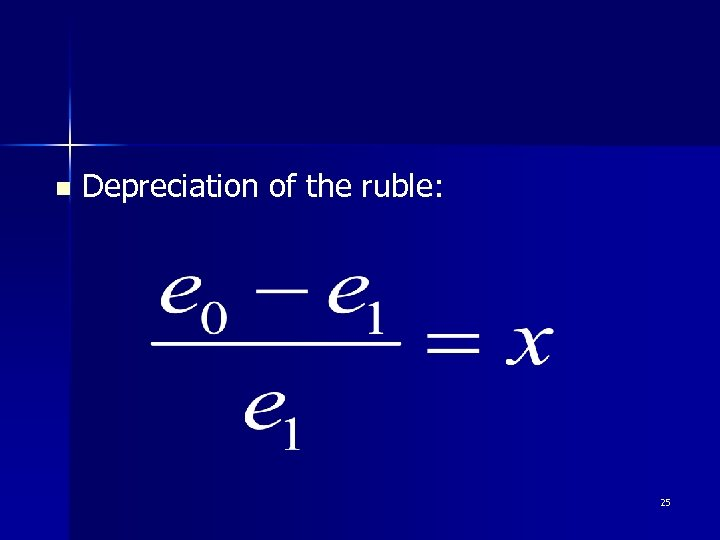n Depreciation of the ruble: 25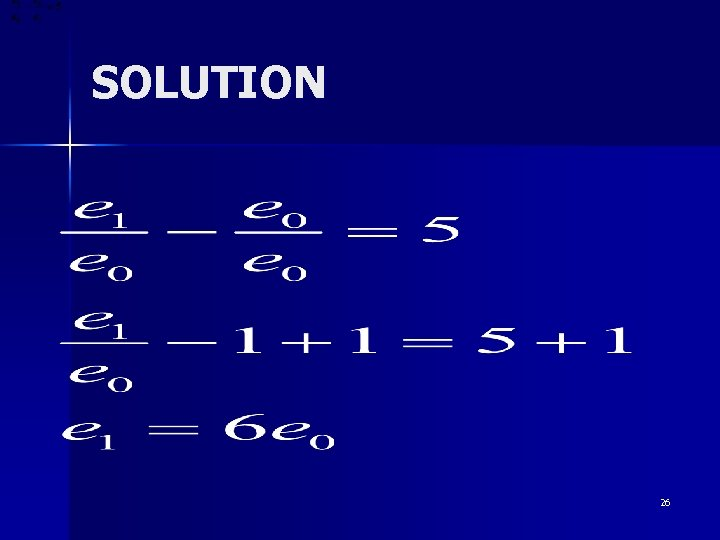SOLUTION 26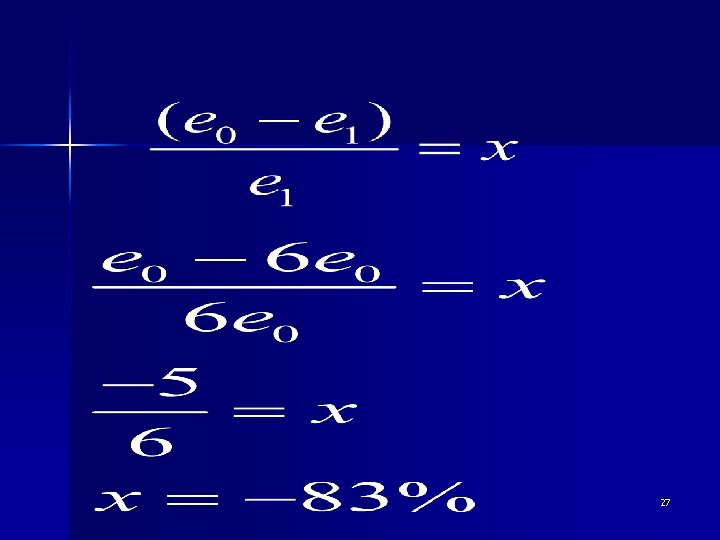27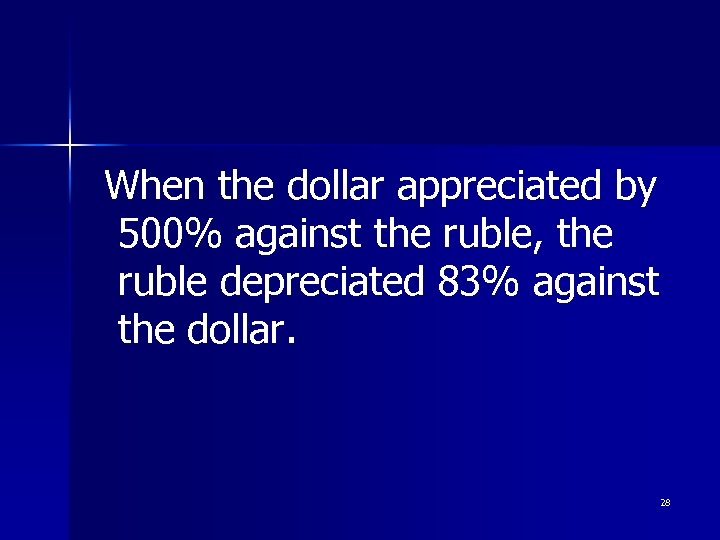When the dollar appreciated by 500% against the ruble, the ruble depreciated 83% against the dollar. 28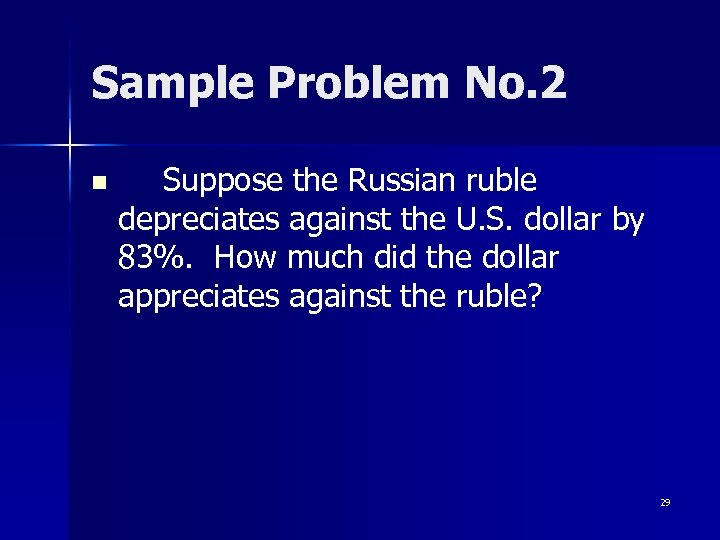Sample Problem No. 2 n Suppose the Russian ruble depreciates against the U. S. dollar by 83%. How much did the dollar appreciates against the ruble? 29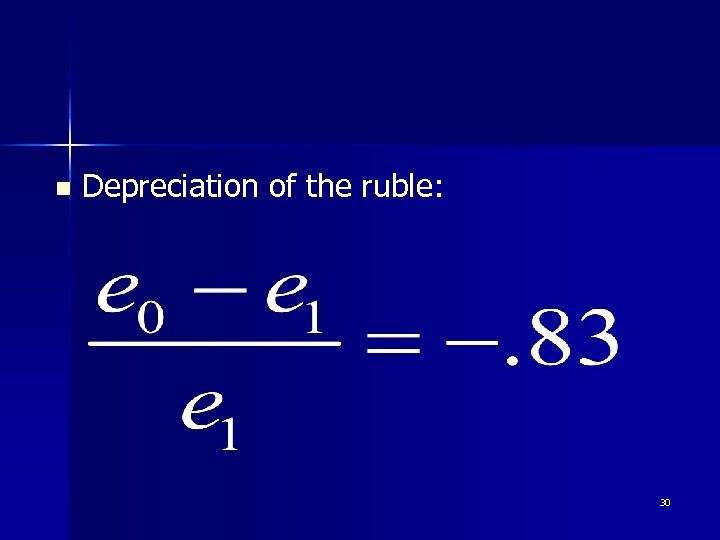n Depreciation of the ruble: 30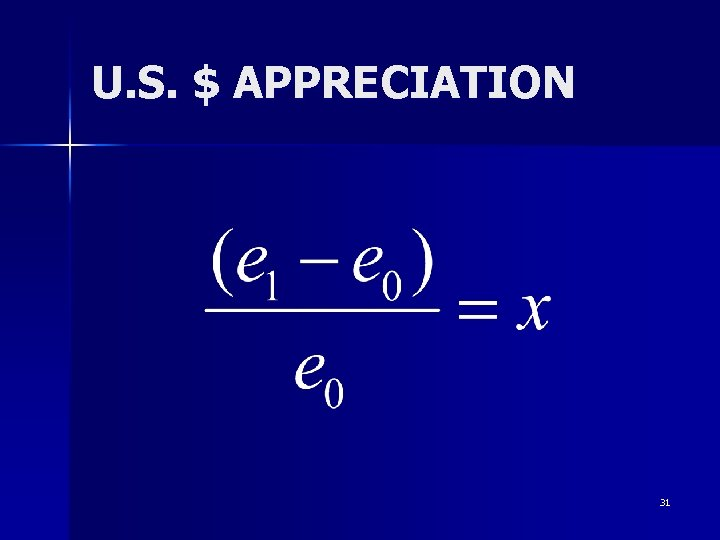U. S. \$ APPRECIATION 31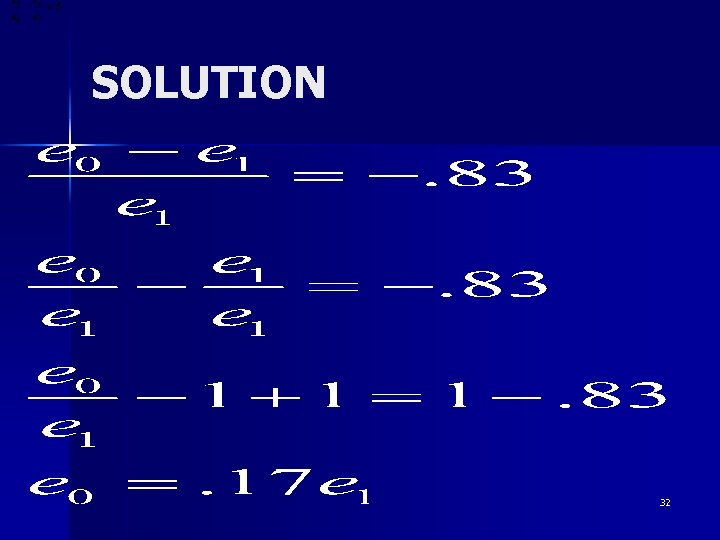SOLUTION 32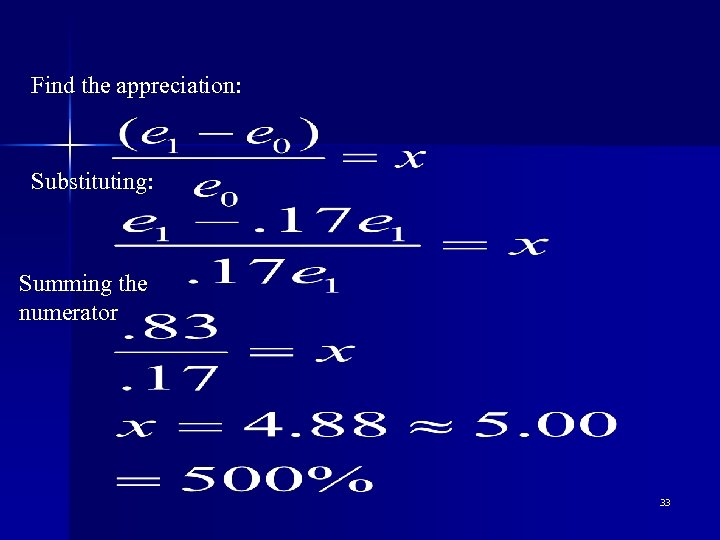Find the appreciation: Substituting: Summing the numerator 33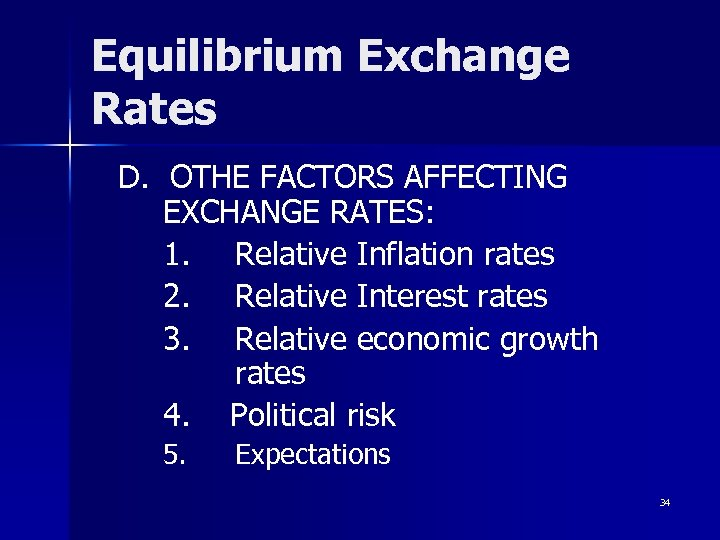Equilibrium Exchange Rates D. OTHE FACTORS AFFECTING EXCHANGE RATES: 1. Relative Inflation rates 2. Relative Interest rates 3. Relative economic growth rates 4. Political risk 5. Expectations 34